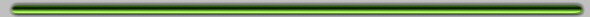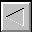Codes/Data of Rennan Barkana# Public codes and data from publications

The list below includes:

INFALL: a code for calculating the mean initial and final density profiles around a virialized dark matter halo.
GEMINI: a toolkit for analytical models of two-point correlations and inhomogeneous structure formation.
FASTELL: a code to calculate lensing by an elliptical galaxy model.
Q0957+561: the full covariance matrix from VLBI fitting of the lensed jets.# INFALL

Infall is a code for calculating the mean initial and final density profiles around a virialized dark matter halo. The initial profile is derived from the statistics of the initial Gaussian random field, accounting for the problem of peaks within peaks using the extended Press-Schechter model. Spherical collapse then yields the typical density and velocity profiles of the gas and dark matter that surrounds the final, virialized halo. In additional to the mean profile, ±1-σ profiles are calculated and can be used as an estimate of the scatter. The code was developed by Rennan Barkana, 2002/3.

The code in C cosistes of three files:
Infall.c (main program)
InfallSub.c (functions and subroutines)
Compile, e.g., with: gcc -O Infall.c -o Infall.out -lm

Here are six example input command files and the resulting output data files (Each file takes a couple seconds to run). These consider a 1010 solar mass halo that virializes at redshift 2. WMAP 5-year cosmological parameters are assumed.
Infall.out < Infall1.in > Infall1.out (mean initial profile)
Infall.out < Infall2.in > Infall2.out (-1-σ initial profile)
Infall.out < Infall3.in > Infall3.out (+1-σ initial profile)
Infall.out < Infall4.in > Infall4.out (mean final profile)
Infall.out < Infall5.in > Infall5.out (-1-σ final profile)
Infall.out < Infall6.in > Infall6.out (+1-σ final profile)

Here are two example figures that use these data files.
Supermongo input: InfallEx1.mon, resulting EPS figure: InfallEx1.eps. This figure is in the style of Figure 3 in Barkana (2004) (but it includes only some of the curves, and the cosmological parameters are slightly different). The same data files can be used to create figures in the style of Figures 1 and 2 from Barkana (2004).
Supermongo input: InfallEx2.mon, resulting EPS figure: InfallEx2.eps. This figure is in the style of Figure 4 in Barkana (2004) (but it includes only one curve, from the middle panel, and the cosmological parameters are slightly different).# GEMINI

Gemini is a toolkit for analytical models of two-point correlations and inhomogeneous structure formation. It extends standard Press-Schechter theory to inhomogeneous situations, allowing a realistic, analytical calculation of halo correlations and bias. The code was developed by Evan Scannapieco and Rennan Barkana, 2000 - 2001.

The paper [ApJ 571, 585 (2002)], entitled "An Analytical Approach to Inhomogeneous Structure Formation", is available on the astro-ph archive, here.

The code in C is available here.# FASTELL

FASTELL is a code to calculate quickly and accurately the lensing deflection and magnification matrix for the SPEMD lens galaxy model. The SPEMD consists of a softened power-law radial distribution with elliptical isodensity contours. The code was developed by Rennan Barkana, Fall 1997 - 1998.

The paper [ApJ 502, 531 (1998)], entitled "Fast calculation of a family of elliptical mass gravitational lens models", is available on the astro-ph archive, here. (417 kbytes)

The same paper but with color figures is available (in postscript) here. (417 kbytes)

The code in Fortran is available here. (163 kbytes)# Q0957+561

We give here the covariance matrix from VLBI fitting by Barkana et al. (1999) of the Garrett et al. (1994) data of Q0957+561.

The paper [ApJ 520, 479 (1999)], entitled "A reassessment of the data and models of the gravitational lens Q0957+561", is available on the astro-ph archive, here. (417 kbytes)

In the paper we increased our parameter error estimates from the following by an additional factor of the square root of the reduced chisq, where the reduced chisq = 2.1933586480011 for 59 parameters fit to a total of 21040 visibility amplitudes and phases.

In the following, we give a complete covariance matrix for 74 parameters (of which 59 are independent). The order is: 34 A component parameters (corresponding to the top half of Table 1 in the paper. Thus there is a Flux, x-comp, y-comp, Maj. Axis, Axis Ratio, and Pos. Ang. for each of six components, except that the position of component #1 is set to 0,0 .), 34 B component parameters (as in the bottom half of Table 1 in the paper), and six magnification parameters (as in Table 2): M1 at A5, M2 at A5, phi_1, phi_2, M1 at A1, M2 at A1.

Click here for a file with the 74 parameters and their one-sigma errors.

Click here for a file with the normalized Covariance Matrix (Order is A_[ij], with j=i to 74 for each of i=1 to 74. Number of entrees is 2775, in 925 lines of 3 numbers each).Back to Homepage